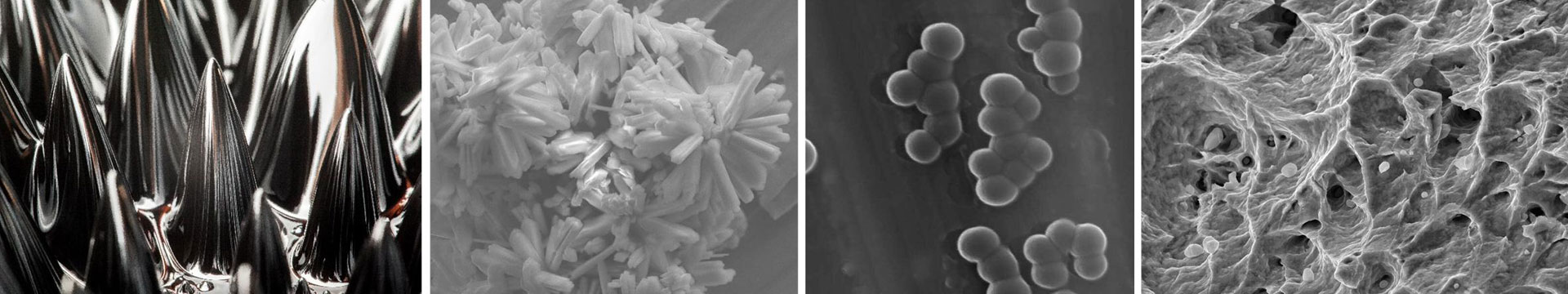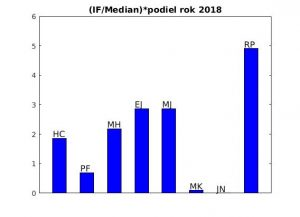•# 2018

## Publikácie pracovníkov oddelenia za rok 2018

1. H. ČENČARIKOVÁ, J. STREČKA, and A. GENDIAR, Magnetization processes and existence of reentrant phase transitions in coupled spin-electron model on doubly decorated planar lattices, Journal of Magnetism and Magnetic Materials 452 (2018) 512  [IF/MedIF(2017)=3.046/2.227=1.368] (10 pages).
2. H. ČENČARIKOVÁ and DOMINIK LEGUT, The effect of relativity on stability of Copernicium phases, their electronic structure and mechanical properties, Physica B 536 (2018) 576  [IF/MedIF(2017)=1.453/2.227=0.652] (7 pages).
3. H. ČENČARIKOVÁ, JOZEF STREČKA, ANDREJ GENDIAR, and NATÁLIA TOMAŠOVIČOVÁ, The influence of further-neighbor spin-spin interaction on a ground state of 2D coupled spin-electron model in a magnetic field, Physica B 536 (2018) 432 [IF/MedIF(2017)=1.453/2.227=0.652] (7 pages).
4. * R. KRČMAR, J. GENZOR, Y. LEE, H. ČENČARIKOVÁ,  T. NISHINO, and ANDREJ GENDIAR, Tensor-network study of a quantum phase transition on the Sierpiński fractal,  Physical Review E 98 (2018) 062114 [IF/MedIF(2017)=2.284/1.306=1.749] (8 pages).
5. * H. ČENČARIKOVÁ and JOZEF STREČKA, Enhanced magnetoelectric effect of the exactly solved spin-electron model on a doubly decorated square lattice in the vicinity of a continuous phase transition, Physical Review E 98 (2018) 062129 [IF/MedIF(2017)=2.284/1.306=1.749] (7 pages).
6. P. FARKAŠOVSKÝ, Effects of geometrical frustration on ferromagnetism in the Hubbard model on the generalised Shastry-Sutherland lattice, The European Physical Journal B 91 (2018) 74   [IF/MedIF(2017)=1.536/2.227=0.690] (6 pages).
7. M. HNATIČ, G. KALAGOV, and N. Yu. NALIMOV, Turbulent mixing of a critical fluid: The non-perturbative renormalization, Nuclear Physics B 926 (2018) 1 [IF/MedIF(2017)=3.285/2.290=1.434] (10 pages).
8. M. HNATIČ, G. KALAGOV, and N. Yu. NALIMOV, 2D Bose condensation and Goldstone singularities,  Nuclear Physics B 936 (2018) 206 [IF/MedIF(2017)=3.285/2.290=1.434] (9 pages).
9. V. ALTAISKY, M. HNATICH, and N. E. KAPUTKINA, Renormalization of viscosity in wavelet-based model of turbulence,  Physical Review E 98 (2018) 033116 [IF/MedIF(2017)=2.284/1.306=1.749] (14 pages).
10. M. HNATIČ, P. ZALOM, Helical turbulent Prandtl number in the A model of passive vector advection: Two loop Approximation, Physics of Atomic Nuclei  (2018) 81 [IF/MedIF(2017)=0.524/1.903=0.275].
11. M. HNATIČ, G.KALAGOV, T. LUČIVJANSKÝ, Scaling behavior in interacting systems: joint effect of anisotropy and compressibility, The European Physical Journal B 91 (2018) 269   [IF/MedIF(2017)=1.536/2.227=0.690] (12 pages).
12. V. M. KHMARA, M. HNATIČ, V. Yu. LAZUR, and O. K. REITY, Quasicrossings of potential curves in the two-Coulomb-center problem, The European Physical Journal D 72 (2018) 39        [IF/MedIF(2017)=1.393/2.278=0.612] (10 pages).
13. E. JURČIŠINOVÁ and M. JURČIŠIN, Antiferromagnetic geometric frustration under the influence of the next-nearest-neighbor interaction. An exactly solvable model,  Physica A 492 (2018) 1798 [IF/MedIF(2017)=2.132/1.652=1.291] (25 pages).
14. E. JURČIŠINOVÁ and M. JURČIŠIN, Highly macroscopically degenerated single-point ground states as source of specific heat capacity anomalies in magnetic frustrated systems, Journal of Magnetism and Magnetic Materials 451 (2018) 137  [IF/MedIF(2017)=3.046/2.227=1.368] (6 pages).
15. A. BOBÁK, E. JURČIŠINOVÁ,  M. JURČIŠIN, and M. ŽUKOVIČ, Frustrated spin-1/2 Ising antiferromagnet on a square lattice in a transverse field, Physical Review E 97 (2018) 022124 [IF/MedIF(2017)=2.284/1.306=1.749] (7 pages).
16.  E. JURČIŠINOVÁ and  M. JURČIŠIN, Multipeak low-temperature behavior of specific heat capacity in frustrated magnetic systems: An exact theoretical analysis, Physical Review E 97 (2018) 052129 [IF/MedIF(2017)=2.284/1.306=1.749](16 pages).
17. * E. JURČIŠINOVÁ,  M. JURČIŠIN, and M. MENKYNA, Anomalous scaling in the Kazantsev-Kraichnan model with finite time correlations: two-loop renormalization group analysis of relevant composite operators, The European Physical Journal B 91 (2018) 313  [IF/MedIF(2017)=1.536/2.227=0.690] (23 pages).
18. M. KUPKOVÁ, M. KUPKA, M. HRUBOVČÁKOVÁ, R. ORIŇÁKOVÁ, A. MOROVSKÁ TUROŇOVÁ, and V. PUCHÝ, Study on Corrosion of Iron-Zinc Oxide Particulate Composites Produced by a Spark Plasma Sintering in Hanks‘ Solution,  Int. J. Electrochem. Sci., 13 (2018) 11839 [IF/MedIF(2017)=1.369/2.492=0.549] (14 pages).
19. A. SEPEHRI and R. PINČÁK, G-theory and its reduction to F(R)-gravity in FRW universe, International Journal of Geometric Methods in Modern Physics 15 (2018) 1850144 [IF/MedIF(2017)=1.009/1.306=0.773] (15 pages).
20. S. CAPOZZIELLO, R. PINČÁK, K. KANJAMAPORNKUL, and E. N. SARIDAKIS, The Chern-Simons Current in Systems of DNA-RNA Transcriptions, Annalen der Physik (2018) 1700271 [IF/MedIF(2017)=2.557/1.652=1.548] (35 pages).
21. S. CAPOZZIELLO, R. PINČÁK, and E. N. SARIDAKIS, Constructing superconductors by graphene Chern–Simons wormholes, Annals of Physics 390 (2018) 303[IF/MedIF(2017)=2.367/1.652=1.433] (31 pages).
22. J. SMOTLACHA and R. PINČÁK, Electronic properties of phosphorene and graphene nanoribbons with edge vacancies in magnetic field, Physics Letters A 382 (2018) 846 [IF/MedIF(2017)=1.863/1.652=1.128] (9 pages).
23. A. SEPEHRI, R. PINČÁKM. HNATIČ, F. RAHAMAN,  and A. PRADHAN, Quarkonium in a thermal BIon, Canadian Journal of Physics 96 (2018) 127 [IF/MedIF(2017)=0.983/1.652=0.595] (21 pages).
24. T. GHAFFARY and  R. PINČÁK, The Information Loss for QCD Matter in Cylindrical Black Holes at LHC, Int. J. Theor. Phys. vol. 57, issue 3 (2018) 898[IF/MedIF(2017)=0.968/1.652=0.586] (11 pages).
25. S. CAPOZZIELLO, O. LUONGO, R. PINČÁK, and A. RAVANPAK, Cosmic acceleration in non-flat f(T) cosmology, General Relativity and Gravitation 50 (2018) 53 [IF/MedIF(2017)=1.721/1.652=1.042] (23 pages).
26. A. SEPEHRI and  R. PINČÁK, G-theory: The generator of M-theory and supersymmetry, Mod. Phys. Lett. A 33 (2018) 1850058 [IF/MedIF(2017)=1.308/1.306=1.002] (23 pages).
27. S. CAPOZZIELLO and R. PINČÁK, The Chern–Simons current in time series of knots and links in proteins, Annals of Physics 393 (2018) 413 [IF/MedIF(2017)=2.367/1.652=1.433] (34 pages).
28. R. PINČÁK, D-brane solutions under market panic, International Journal of Geometric Methods in Modern Physics 15 (2018) 1850099 [IF/MedIF(2017)=1.009/1.306=0.773] (23 pages).
29. R. PINČÁK and K. KANJAMAPORNKUL, Model of the Financial Market with the Minkowski Metric, Zeitschrift für Naturforschung A  73 (2018) 669 [IF/MedIF(2017)=1.414/1.652=0.856] (16 pages).### Publikácie v registrovaných zahraničných karentovaných neimpaktovaných časopisoch a zborníkoch:

1. M. HNATIČ, V. M. KHMARA, V. Yu. LAZUR, and O. K. REITY, Splitting of Potential Curves in the Two-Coulomb-Centre Problem, EPJ Web of Conferences 173 (2018) 02008 (4 pages).
2. L. Ts. ADZHEMYAN, M. HNATIČ, M. V. KOMPANIETS, T. LUČIVJANSKÝ, and L. MIŽIŠIN, Directed Percolation: Calculation of Feynman Diagrams in the Three-Loop Approximation, EPJ Web of Conferences 173 (2018) 02001 (4 pages).
3. E. JURČIŠINOVÁ, M. JURČIŠIN, and R. REMECKY, Diffusion Processes in the A-Model of Vector Admixture: Turbulent Prandtl Number, EPJ Web of Conferences 173 (2018) 02009 (4 pages).

### Publikácie v registrovaných zahraničných nekarentovaných neimpaktovaných časopisoch a zborníkoch:

1. V. V. SHUNAEV, R. PINČÁK, and J. SMOTLACHA, Novaya metodika roscheta energeticheskoy shcheli bisloynich fullerenov, VOPROSY PRIKLADNOY FIZIKI 25 (2018) 66 (5 pages).

### Publikácie v domácich nekarentovaných neimpaktovaných časopisoch a zborníkoch:

1. M. KUPKOVÁ, M. HRUBOVČÁKOVÁ, M. KABÁTOVÁ, and M. KUPKA, Microstructure, Mechanical Properties and Corrosion Behaviour of Iron-Manganese PM Materials Pressed and Sintered Once and Twice, Powder Metallurgy Progress, 17 (2017) 112 (12 pages).